# Matplotlib: Pyplot By Example

Last updated:

Pyplot or Matplotlib? What's The difference?

All examples can be found online on this jupyter notebook

## Change Figure size

After plotting, get a reference to the current figure and call set_size_inches(width,height):

import numpy as np
import matplotlib.pyplot as plt

x = np.linspace(0.0,100,50)
y = np.random.uniform(low=0,high=10,size=50)

plt.scatter(x,y)

# get reference to the current figure
fig = plt.gcf()
fig.set_size_inches(8,3)

plt.show()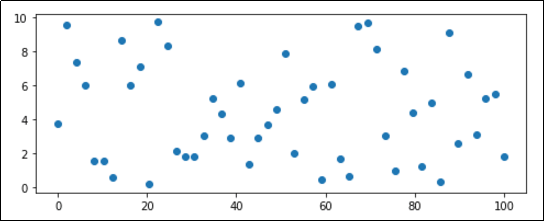Customized image with 8x3 inches.
(Default size is 6x4)

## Save plot to file

Use plt.savefig().

The image format is deduced from the extension ('png', 'jpg', 'svg', etc)

import numpy as np
import matplotlib.pyplot as plt

x = np.linspace(0.0,100,50)
y = np.random.uniform(low=0,high=10,size=50)

plt.scatter(x,y)

plt.savefig('out.png')


## Multiple subplots in Figure

View Matplotlib Subplots: Best Practices and Examples more multiple subplot examples

Call plt.subplots() to get a figure reference and individual Axes references (one for each subplot)

import numpy as np
import matplotlib.pyplot as plt

x = np.linspace(0.0,100,50)
y = np.random.uniform(low=0,high=10,size=50)

# passing 2,2 as parameters indicates that you will
# get 4 subplots (2 rows and 2 columns)
fig, axes = plt.subplots(2,2)

# just plot things on each individual axes

axes.scatter(x,y,c='red',marker='+')

axes.bar(x,y)

axes.scatter(x,y,marker='x')

axes.barh(x,y)
# you can set the title for a single subplot
axes.set_title('Plot 4',size=14)

plt.show()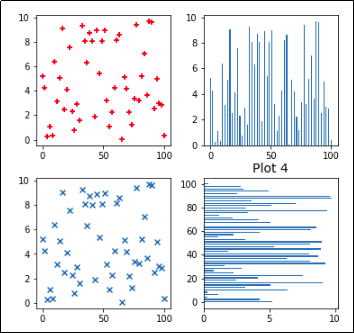Create a figure with 4 individual subplots using plt.subplots().
(Note that only Plot 4 has had its Title set.)

View Matplotlib Subplots: Best Practices and Examples more multiple subplot examples

To adjust the padding, the space between the subplots in a single Figure, use plt.subplots_adjust()

import numpy as np
import matplotlib.pyplot as plt

x = np.linspace(0.0,100,50)
y = np.random.uniform(low=0,high=10,size=50)

# passing 2,2 as parameters indicates that you will
# get 4 subplots (2 rows and 2 columns)
fig, axes = plt.subplots(2,2)

# just plot things on each individual axes
axes.scatter(x,y,c='red',marker='+')
axes.bar(x,y)
axes.scatter(x,y,marker='x')
axes.barh(x,y)

# here, set the width and the height between the subplots
# the default value is 0.2 for each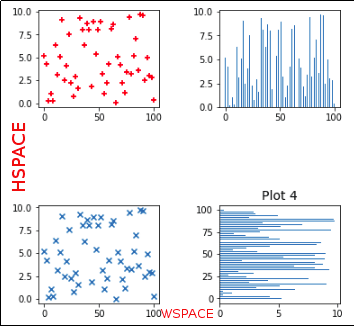Set wspace to 0.50 and hspace to 1.0 to
increase the width and the height,
respectively.

## Set Figure Title, Font size

import numpy as np
import matplotlib.pyplot as plt

x = np.linspace(0.0,100,50)
y = np.random.uniform(low=0,high=10,size=50)

plt.scatter(x,y)

# get reference to the current figure
fig = plt.gcf()

# HERE
fig.suptitle('IMAGE TITLE HERE', fontsize=18)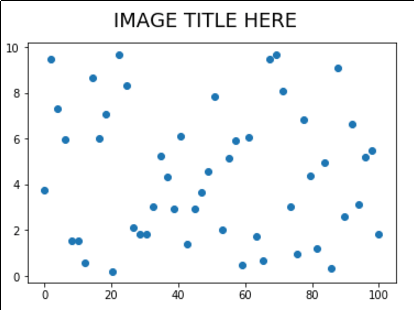suptitle() acceps parameters you would normally use in matplotlib.Text

## Set Axis Title, Font size

Similar to the above, but acts on a single Axis (useful if you have multiple suplots on the same Figure)

import numpy as np
import matplotlib.pyplot as plt

x = np.linspace(0.0,100,50)
y = np.random.uniform(low=0,high=10,size=50)

plt.scatter(x,y)

# get reference to the current axis
ax = plt.gca()

# HERE
ax.set_title('title for this axis only', fontsize=20)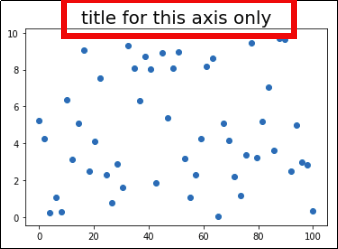If there were multiple axis in a
single Figure, only that Axis would have
its Title set

## Tick label rotation

Use plt.xticks() or plt.yticks() and set the rotation argument (degrees)

If you want to call this on an Axes object instead, do: ax.set_xticklabels(ax.get_xticklabels(),rotation=60)

import numpy as np
import matplotlib.pyplot as plt

x = np.linspace(0.0,100,50)
y = np.random.uniform(low=0,high=10,size=50)

plt.plot(x,y)

# rotating labels on the xaxis
plt.xticks(rotation=60)
# y axis
plt.yticks(rotation=60)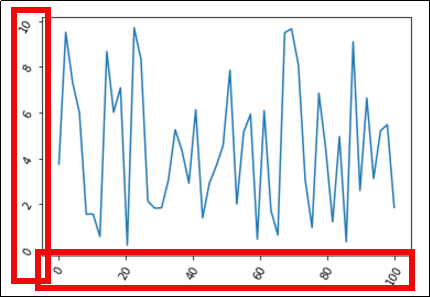Change the label rotation in 60 degs for both the x-axis
and the y-axis with plt.xticks(60) and plt.yticks(60)

## Set Axis labels, Font size

Use plt.xlabel() or plt.ylabel, using the same arguments that are accepted by plt.text().

import numpy as np
import matplotlib.pyplot as plt

x = np.linspace(0.0,100,50)
y = np.random.uniform(low=0,high=10,size=50)

plt.plot(x,y)

# HERE
plt.xlabel('time (s)',color='red',fontsize=30)
plt.ylabel('temperature (C)', fontsize=15)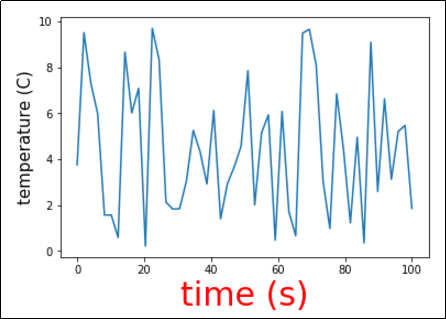You can use any other arguments from plt.text() too.

## Set y-axis, x-axis limits

import numpy as np
import matplotlib.pyplot as plt

x = np.linspace(0.0,100,50)
y = np.random.uniform(low=0,high=10,size=50)

# for the whole plot
plt.plot(x,y)

# HERE
plt.ylim(-5,15)
plt.xlim(-30,130)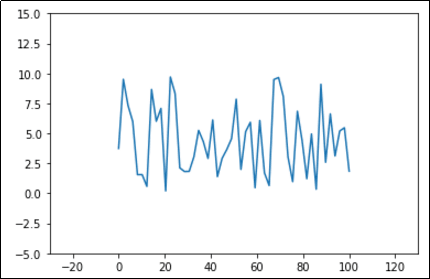Same data as before, with more room around it.

## Label points in plot

This was inspired by a guy on stackoverflow but I can't remember. If you know please send a message.

First define a function called plot_value_labels() and call it on your axis:

def plot_value_labels(axis):
rects = axis.patches

# For each bar: Place a label
for rect in rects:
# Get X and Y placement of label from rect.
y_value = rect.get_height()
x_value = rect.get_x() + rect.get_width() / 2

label = '{:.2f}'.format(y_value)

# Vertical alignment for positive values
va = 'bottom'

# If value of bar is negative: Place label below bar
if y_value < 0:
# Invert space to place label below
space *= -1
# Vertically align label at top
va = 'top'

# Create annotation here
axis.annotate(label, (x_value, y_value),
xytext=(0, 2),
textcoords="offset points",
ha='center',
rotation=45,
va=va)

# now the actual code
import matplotlib.pyplot as plt
import numpy as np

# generate sample data for this example
x = np.linspace(0.0,10,10)
y = np.random.uniform(low=0,high=6,size=10)

# plot bar plot
plt.bar(x,y)

# call the function we defined
plot_value_labels(plt.gca())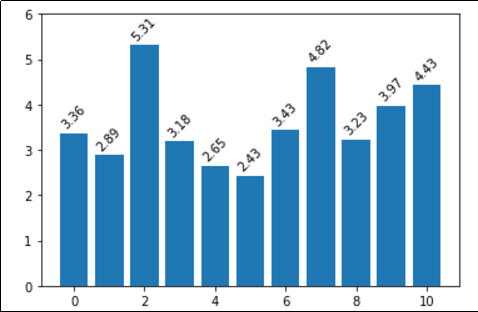Simple labels on top of bars in a bar plot

## Set tick frequency

Use plt.xticks with np.arange (for the y-axis, use yticks instead).

Template: plt.xticks(np.arange(<start>,<end>,<step>)).

import numpy as np
import matplotlib.pyplot as plt

# generate sample data for this example
x = np.linspace(0.0,100,50)
y = np.random.uniform(low=0,high=10,size=50)

plt.plot(x,y)

# HERE tell pyplot to write a x-axis tick every 5 units
plt.xticks(np.arange(0, 100, 5))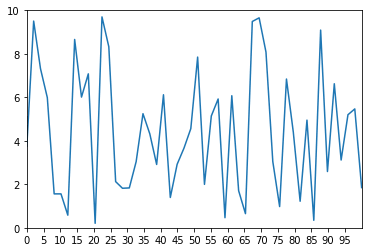X-Axis ticks are drawn every 5 units, as per the call to xticks

All options available to Line2D can be passed to the grid() function.

Use ax.grid(True) on the axis object

To add grid for the whole figure instead, simple use plt.grid(True)

import matplotlib.pyplot as plt
import numpy as np

# generate sample data for this example
x = np.linspace(0.0,100,50)
y = np.random.uniform(low=0,high=10,size=50)

# HERE linewidth and linestyle are some of the options you can set
# gca means Get Current Axis
plt.gca().grid(True, linewidth=0.7, linestyle=':')

# then plot the chart as you would normally
plt.plot(x,y)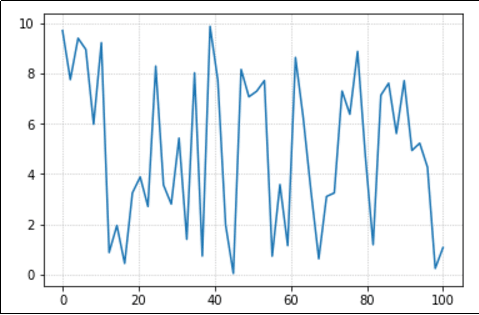0.7 is a reasonable setting for the line width

## Histogram, numpy array

View all API options: pyplot docs: pyplot.hist

import matplotlib.pyplot as plt
import numpy as np

# generate sample data following a normal distribution
values = np.random.normal(size=100)
# array([ 0.49671415, -0.1382643 ,  0.64768854,...

# see all examples in the API link
plt.hist(values,rwidth=0.9,bins=[-3,-2,-1,0,1,2,3])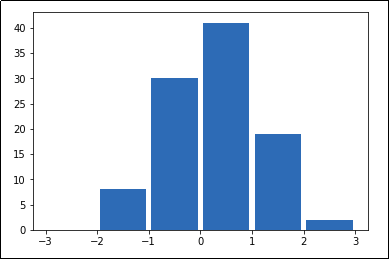A histogram is a simple chart that shows how frequent
each value is. It shows the discrete distribution of values in
a collection.

## String axis labels, bar plot

Call plt.xticks(x_values, labels):

import matplotlib.pyplot as plt
import numpy as np

# generate sample data for this example
xs = [1,2,3,4,5,6,7,8,9,10,11,12]
ys = np.random.normal(loc=3.0,size=12)
labels = ['jan','feb','mar','apr','may','jun','jul','aug','sept','oct','nov','dec']

plt.bar(xs,ys)

# HERE tell pyplot which labels correspond to which x values
plt.xticks(xs,labels)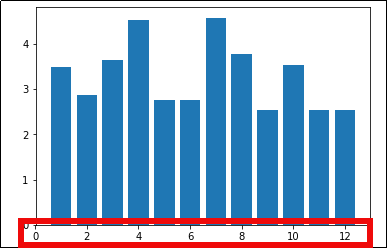If you don't set labels,
each label is the value itself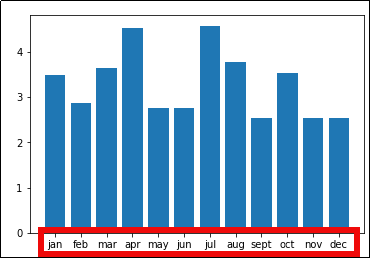Set string labels using plt.xticks(xs,labels)

## String axis labels, horizontal bar plot

import matplotlib.pyplot as plt
import numpy as np

# generate sample data for this example
xs = [1,2,3,4,5,6,7,8,9,10,11,12]
ys = np.random.normal(loc=3.0,size=12)
labels = ['jan','feb','mar','apr','may','jun','jul','aug','sept','oct','nov','dec']

plt.barh(xs,ys)

# HERE tell pyplot which labels correspond to which x values
plt.yticks(xs,labels)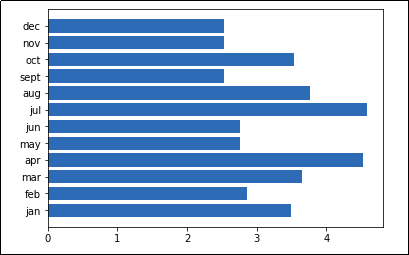Horizontal bar plots are a useful alternative
when there is not enough space for labels
on the x (horizontal) axis

## Twin plots example

To create a figure with a second y-axis in the same plot, use ax.twinx():

import matplotlib.pyplot as plt
import numpy as np

plt.clf()

# generate sample data for this example
xs = [1,2,3,4,5,6,7,8,9,10,11,12]
ys_bars = np.random.normal(loc=3.0,size=12)
ys_lines = np.random.normal(loc=5.0,size=12,scale=0.5)

# this is the axis on the left
ax1=plt.gca()
ax1.bar(xs,ys_bars,color='green')

# order is important when setting ticks.
# Ticks must be set after the plot has been drawn
ax1.set_yticks(np.arange(0,11,1))
ax1.set_yticklabels(np.arange(0,11,1),color='green')

# create the 'twin' axis on the right
ax2=ax1.twinx()

# plot the same numbers but multiplied by 20
ax2.plot(xs,ys_lines*20,color='red')

# set the ticks and ticklabels for the twin axis
ax2.set_yticks(np.arange(0,101,10))
ax2.set_yticklabels(np.arange(0,101,10),color='red')

# set ticks for the common x axis (bottom)
ax2.xaxis.set_ticks(xs)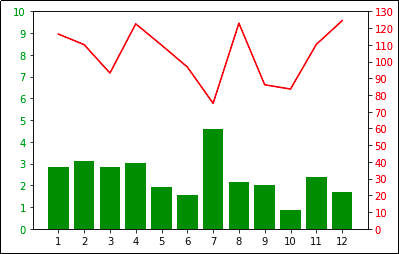Plot two different series on the same graph.
Note that each axis has a different scale.

## Align axis labels on twin axes

Use ax.yaxis.set_major_locator(LinearLocator(numticks)) on both the original and the twin axis, with the same number of ticks.

import matplotlib.pyplot as plt
import numpy as np

plt.clf()

# generate sample data for this example
xs = [1,2,3,4,5,6,7,8,9,10,11,12]
ys_bars = np.random.normal(loc=3.0,size=12)
ys_lines = np.random.normal(loc=5.0,size=12,scale=0.5)

# this is the axis on the left
ax1=plt.gca()

ax1.bar(xs,ys_bars,color='green')

# order is important when setting ticks.
# Ticks must be set after the plot has been drawn
ax1.set_yticks(np.arange(0,11,1))

# define the number of ticks
NUM_TICKS=11

# change the tick locator for this axis and set the desired number of ticks
ax1.yaxis.set_major_locator(plt.LinearLocator(numticks=NUM_TICKS))

# create the 'twin' axis on the right
ax2=ax1.twinx()

# plot the same numbers but multiplied by 20
ax2.plot(xs,ys_lines*20,color='red')

# set the ticks for the twin axis
ax2.set_yticks(np.arange(0,101,10))

# change the tick locator for this axis and set the desired number of ticks
ax2.yaxis.set_major_locator(plt.LinearLocator(numticks=NUM_TICKS))

# set ticks for the common x axis (bottom)
ax2.xaxis.set_ticks(xs)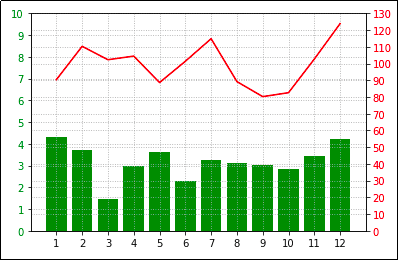Since each axis has a different
make things look ugly.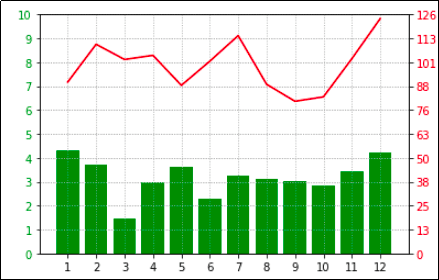You can align the scales using
plt.LinearLocator() to set the number
of ticks you want in both scales.

## Custom color cycle

If you wish to override the default colours used by pyplot (for example, to make it easier to colourblind people to view your images), you can use set_prop_cycle() on an Axes instance:

import numpy as np
import matplotlib.pyplot as plt

# generate random data for plotting
x = np.linspace(0.0,100,50)
y2 = x*2
y3 = x*3
y4 = x*4
y5 = x*5
y6 = x*6

# plot multiple lines in the same plot
plt.plot(x,y2,label='y=2x')
plt.plot(x,y3,label='y=3x')
plt.plot(x,y4,label='y=4x')
plt.plot(x,y5,label='y=5x')
plt.plot(x,y6,label='y=6x')

colour_blind_cycle = ['#377eb8', '#ff7f00', '#4daf4a',
'#f781bf', '#a65628', '#984ea3',
'#999999', '#e41a1c', '#dede00']

plt.gca().set_prop_cycle(color=colour_blind_cycle)

plt.legend()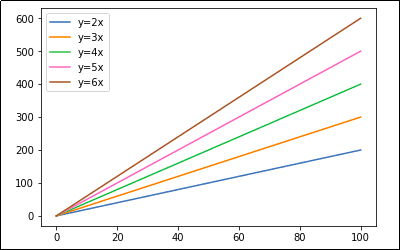A simple line plot using a colourblind-friendly color scheme
(Props to user thriveth on Github.)